Circular Motion and Satellite Motion - Lesson 2 - Applications of Circular Motion

# Roller Coasters and Amusement Park Physics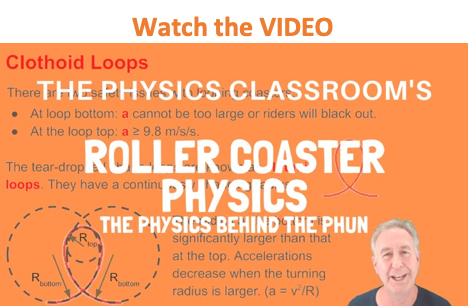People are wild about amusement parks. Each day, we flock by the millions to the nearest park, paying a sizable hunk of money to wait in long lines for a short 60-second ride on our favorite roller coaster. The thought prompts one to consider what is it about a roller coaster ride that provides such widespread excitement among so many of us and such dreadful fear in the rest? Is our excitement about coasters due to their high speeds? Absolutely not! In fact, it would be foolish to spend so much time and money to ride a selection of roller coasters if it were for reasons of speed. It is more than likely that most of us sustain higher speeds on our ride along the interstate highway on the way to the amusement park than we do once we enter the park. The thrill of roller coasters is not due to their speed, but rather due to their accelerations and to the feelings of weightlessness and weightiness that they produce. Roller coasters thrill us because of their ability to accelerate us downward one moment and upwards the next; leftwards one moment and rightwards the next. Roller coasters are about acceleration; that's what makes them thrilling. And in this part of Lesson 2, we will focus on the centripetal acceleration experienced by riders within the circular-shaped sections of a roller coaster track. These sections include the clothoid loops (that we will approximate as a circle), the sharp 180-degree banked turns, and the small dips and hills found along otherwise straight sections of the track.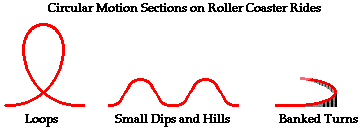### The Physics of Roller Coaster Loops

The most obvious section on a roller coaster where centripetal acceleration occurs is within the so-called clothoid loops. Roller coaster loops assume a tear-dropped shape that is geometrically referred to as a clothoid. A clothoid is a section of a spiral in which the radius is constantly changing. Unlike a circular loop in which the radius is a constant value, the radius at the bottom of a clothoid loop is much larger than the radius at the top of the clothoid loop. A mere inspection of a clothoid reveals that the amount of curvature at the bottom of the loop is less than the amount of curv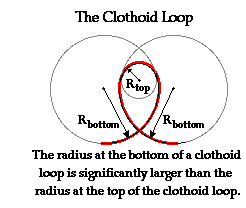ature at the top of the loop. To simplify our analysis of the physics of clothoid loops, we will approximate a clothoid loop as being a series of overlapping or adjoining circular sections. The radius of these circular sections is decreasing as one approaches the top of the loop. Furthermore, we will limit our analysis to two points on the clothoid loop - the top of the loop and the bottom of the loop. For this reason, our analysis will focus on the two circles that can be matched to the curvature of these two sections of the clothoid. The diagram at the right shows a clothoid loop with two circles of different radius inscribed into the top and the bottom of the loop. Note that the radius at the bottom of the loop is significantly larger than the radius at the top of the loop.

As a roller coaster rider travels through a clothoid loop, she experiences an acceleration due to both a change in speed and a change in direction. A rightward moving rider gradually becomes an upward moving rider, then a leftward moving rider, then a downward moving rider, before finally becoming a rightward-moving rider once again. There is a continuous change in the direction of the rider as she moves through the clothoid loop. And as learned in Lesson 1, a change in direction is one characteristic of an accelerating object. In addition to changing directions, the rider also changes speed. As the rider begins to ascend (climb upward) the loop, she begins to slow down. As energy principles would suggest, an increase in height (and in turn an increase in potential energy) results in a decrease in kinetic energy and speed. And conversely, a decrease in height (and in turn a decrease in potential energy) results in an increase in kinetic energy and speed. So the rider experiences the greatest speeds at the bottom of the loop - both upon entering and leaving the loop - and the lowest speeds at the top of the loop.This change in speed as the rider moves through the loop is the second aspect of the acceleration that a rider experiences. For a rider moving through a circular loop with a constant speed, the acceleration can be described as being centripetal or towards the center of the circle. In the case of a rider moving through a noncircular loop at non-constant speed, the acceleration of the rider has two components. There is a component that is directed towards the center of the circle (ac) and attributes itself to the direction change; and there is a component that is directed tangent (at) to the track (either in the opposite or in the same direction as the car's direction of motion) and attributes itself to the car's change in speed. This tangential component would be directed opposite the direction of the car's motion as its speed decreases (on the ascent towards the top) and in the same direction as the car's motion as its speed increases (on the descent from the top). At the very top and the very bottom of the loop, the acceleration is primarily directed towards the center of the circle. At the top, this would be in the downward direction and at the bottom of the loop it would be in the upward direction.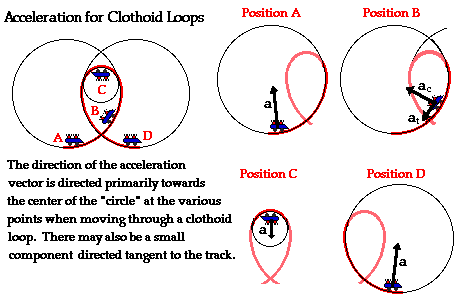### Force Analysis of a Coaster Loop

We learned in Lesson 1 that the inwards acceleration of an object is caused by an inwards net force. Circular motion (or merely motion along a curved path) requires an inwards component of net force. If all the forces that act upon the object were added together as vectors, then the net force would be directed inwards. Neglecting friction and air resistance, a roller coaster car will experience two forces: the force of gravity (Fgrav) and the normal force (Fnorm). The normal force is directed in a direction perpendicular to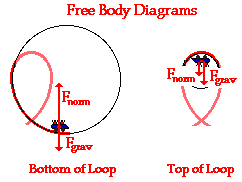the track and the gravitational force is always directed downwards. We will concern ourselves with the relative magnitude and direction of these two forces for the top and the bottom of the loop. At the bottom of the loop, the track pushes upwards upon the car with a normal force. However, at the top of the loop the normal force is directed downwards; since the track (the supplier of the normal force) is above the car, it pushes downwards upon the car. The free-body diagrams for these two positions are shown in the diagrams at the right.

The magnitude of the force of gravity acting upon the passenger (or car) can easily be found using the equation Fgrav = m•g where g = acceleration of gravity (9.8 m/s2). The magnitude of the normal force depends on two factors - the speed of the car, the radius of the loop and the mass of the rider. As depicted in the free body diagram, the magnitude of Fnorm is always greater at the bottom of the loop than it is at the top. The normal force must always be of the appropriate size to combine with the Fgrav in such a way to produce the required inward or centripetal net force. At the bottom of the loop, the Fgrav points outwards away from the center of the loop. The normal force must be sufficiently large to overcome this Fgrav and supply some excess force to result in a net inward force. In a sense, Fgrav and Fnorm are in a tug-of-war; and Fnorm must win by an amount equal to the net force. At the top of the loop, both Fgrav and Fnorm are directed inwards. The Fgrav is found in the usual way (using the equation Fgrav = m•g). Once more the Fnorm must provide sufficient force to produce the required inward or centripetal net force.Earlier in Lesson 2, the use of Newton's second law and free-body diagrams to solve circular motion diagrams was illustrated. It was emphasized at that time that any given physical situation could be analyzed in terms of the individual forces that are acting upon an object. These individual forces must add up as vectors to the net force. Furthermore, the net force must be equal to the mass times the acceleration. The process of conducting a force analysis of a physical situation was first introduced in Unit 2 of The Physics Classroom. Now we will investigate the use of these fundamental principles in the analysis of situations involving the motion of objects in circles. We will utilize the basic problem-solving approach that was introduced earlier in Lesson 2. This approach can be summarized as follows.

 Suggested Method of Solving Circular Motion Problems From the verbal description of the physical situation, construct a free-body diagram. Represent each force by a vector arrow and label the forces according to type. Identify the given and the unknown information (express in terms of variables such as m= , a= , v= , etc.). If any of the individual forces are directed at angles to the horizontal and vertical, then use vector principles to resolve such forces into horizontal and vertical components. Determine the magnitude of any known forces and label on the free-body diagram. (For example, if the mass is given, then the Fgrav can be determined. And as another example, if there is no vertical acceleration, then it is known that the vertical forces or force components balance, allowing for the possible determination of one or more of the individual forces in the vertical direction.) Use circular motion equations to determine any unknown information. (For example, if the speed and the radius are known, then the acceleration can be determined. And as another example, if the period and radius are known, then the acceleration can be determined.) Use the remaining information to solve for the requested information. If the problem requests the value of an individual force, then use the kinematic information (R, T and v) to determine the acceleration and the Fnet; then use the free-body diagram to solve for the individual force value. If the problem requests the value of the speed or radius, then use the values of the individual forces to determine the net force and acceleration; then use the acceleration to determine the value of the speed or radius.

Combine a force analysis with the above method to solve the following roller coaster problem.

 Sample Roller Coaster Problem Anna Litical is riding on The Demon at Great America. Anna experiences a downward acceleration of 15.6 m/s2 at the top of the loop and an upward acceleration of 26.3 m/s2 at the bottom of the loop. Use Newton's second law to determine the normal force acting upon Anna's 864 kg roller coaster car.

Steps 1 and 2 involve the construction of a free body diagram and the identification of known and unknown quantities. This is shown in below.Given Info: m = 864 kg atop = 15.6 m/s2 , down bottom = 26.3 m/s2 , up Find: Fnorm at top and bottom

Step 3 of the suggested method would not apply to this problem since there are no forces directed "at angles" (that is, all the forces are either horizontally or vertically directed). Step 4 of the suggested method involves the determination of any known forces. In this case, the force of gravity can be determined from the equation Fgrav = m • g. Using a g value of 9.8 m/s2, the force of gravity acting upon the 864-kg car is approximately 8467 N. Step 5 of the suggested method would be used if the acceleration were not given. In this instance, the acceleration is known. If the acceleration were not known, then it would have to be calculated from speed and radius information.

Step 6 of the suggested method involves the determination of an individual force - the normal force. This will involve a two-step process: first the net force (magnitude and direction) must be determined; then the net force must be used with the free body diagram to determine the normal force. This two-step process is shown below for the top and the bottom of the loop.

 Bottom of Loop Fnet = m * a Fnet = (864 kg) * (26.3 m/s2, up) Fnet = 22 723 N, up From FBD: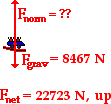Fnorm must be greater than the Fgrav by 22723 N in order to supply a net upwards force of 22723 N. Thus, Fnorm = Fgrav + Fnet Fnorm = 31190 N Top of Loop Fnet = m * a Fnet = (864 kg) * (15.6 m/s2, down) Fnet = 13478 N, down From FBD: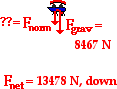Fnorm and Fgrav together must combine together (i.e., add up) to supply the required inwards net force of 13478 N. Thus, Fnorm = Fnet - Fgrav Fnorm = 5011 N

### Sensations of Weightlessness

Observe that the normal force is greater at the bottom of the loop than it is at the top of the loop. This becomes a reasonable fact when circular motion principles are considered. At all points along the loop - which we will refer to as circular in shape - there must be some inward component of net force. When at the top of the loop, the gravitational force is directed inwards (down) and so there is less of a need for a normal force in order to meet the net centripetal force requirement. When at the bottom of the loop, the gravitational force is directed outwards (down) and so now there is a need for a large upwards normal force in order to meet the centripetal force requirement. This principle is often demonstrated in a physics class using a bucket of water tied to a string. The water is spun in a vertical circle. As the water traces out its circular path, the tension in the string is continuously changing. The tension force in this demonstration is analogous to the normal force for a roller coaster rider. At the top of the vertical circle, the tension force is very small; and at the bottom of the vertical circle, the tension force is very large. (You might try this activity yourself outside with a small plastic bucket half-filled with water. Give extra caution to stay clear of all people, windows, trees and overhead power lines. Repeat enough cycles to observe the noticeable difference in tension force when the bucket is at the top and the bottom of the circle.)

If you have ever been on a roller coaster ride and traveled through a loop, then you have likely experienced this small normal force at the top ofthe loop and the large normal force at the bottom of the loop. The normal force provides a feel for a person's weight. (As will be discussed later in Lesson 4, we can never feel our weight; we can only feel other forces that act as a result of contact with other objects.) The more you weigh, the more normal force that you will experience when at rest in your seat. But if you board a roller coaster ride and accelerate through circles (or clothoid loops), then you will feel a normal force that is constantly changing and different from that which you are accustomed to. This normal force provides a sensation or feeling of weightlessness or weightiness. When at the top of the loop, a rider will feel partially weightless if the normal forces become less than the person's weight. And at the bottom of the loop, a rider will feel very "weighty" due to the increased normal forces. It is important to realize that the force of gravity and the weight of your body are not changing. Only the magnitude of the supporting normal force is changing! (The phenomenon of weightlessness will be discussed in much more detail later in Lesson 4.)

There is some interesting history (and physics) behind the gradual usage of clothoid loops in roller coaster rides. In the early days of roller coaster loops, circular loops were used. There were a variety of problems, some of which resulted in fatalities, as the result of the use of these circular loops. Coaster cars entering circular loops at high speeds encountered excessive normal forces that were capable of causing whiplash and broken bones. Efforts to correct the problem by lowering entry speeds resulted in the inability of cars to make it around the entire loop without falling out of the loop when reaching the top. The decrease in speeds as the cars ascended the large circular loop resulted in coaster cars turning into projectile cars (a situation known to be not good for business). The solution to the problem involved using low entry speeds and a loop with a sharper curvature at the top than at the bottom. Since clothoid loops have a continually changing radius, the radius is large at the bottom of the loop and shortened at the top of the loop. The result is that coaster cars can enter the loops at high speeds; yet due to the large radius, the normal forces do not exceed 3.5 G's. At the top of the loop, the radius is small thus allowing a lower speed car to still maintain contact with the track and successfully make it through the loop. The clothoid loop is a testimony to an engineer's application of the centripetal acceleration equation - a = v2/R. Now that's physics for better living!

### Physics of Coaster Dips and Hills

The above discussion and force analysis applies to the circular-like motion of a roller coaster car in a clothoid loop. The second section along a roller coaster track where circular motion is experienced is along the small dips and hills. These sections of track are often found near the end of a roller coaster ride and involve a series of small hills followed by a sharp drop. Riders often feel heavy as they ascend the hill (along regions A and E in the diagram below). Then near the crest of the hill (regions B and F), their upward motion makes them feel as though they will fly out of the car; often times, it is only the safety belt that prevents such a mishap. As the car begins to descend the sharp drop, riders are momentarily in a state of free fall (along regions C and G in the diagram below). And finally as they reach the bottom of the sharp dip (regions D and H), there is a large upwards force that slows their downward motion. The cycle is often repeated mercilessly, churning the riders' stomachs and mixing the afternoon's cotton candy into a slurry of ... . These small dips and hills combine the physics of circular motion with the physics of projectiles in order to produce the ultimate thrill of acceleration - rapidly changing magnitudes and directions of acceleration. The diagram below shows the various directions of accelerations that riders would experience along these hills and dips.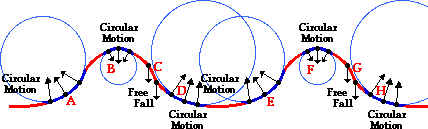### Force Analysis of Coaster Hills

At various locations along these hills and dips, riders are momentarily traveling along a circular shaped arc. The arc is part of a circle - these circles have been inscribed on the above diagram in blue. In each of these regions there is an inward component of acceleration (as depicted by the black arrows). This inward acceleration demands that there also be a force directed towards the center of the circle. In region A, the centripetal force is supplied by the track pushing normal to the track surface. Along region B, the centripetal force is supplied by the force of gravity and possibly even the safety mechanism/bar. At especially high speeds, a safety bar must supply even extra downward force in order to pull the riders downward and supply the remaining centripetal force required for circular motion. There are also wheels on the car that are usually tucked under the track and pulled downward by the track. Along region D, the centripetal force is once more supplied by the normal force of the track pushing upwards upon the car.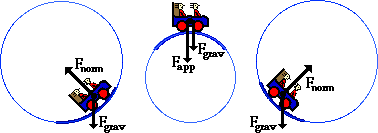The magnitude of the normal forces along these various regions is dependent upon how sharply the track is curved along that region (the radius of the circle) and the speed of the car. These two variables affect the acceleration according to the equation

a = v2 / R

and in turn affect the net force. As suggested by the equation, a large speed results in a large acceleration and thus increases the demand for a large net force. And a large radius (gradually curved) results in a small acceleration and thus lessens the demand for a large net force. The relationship between speed, radius, acceleration, mass and net force can be used to determine the magnitude of the seat force (i.e., normal force) upon a roller coaster rider at various sections of the track. The sample problem below illustrates these relationships. In the process of solving the problem, the same problem-solving strategy enumerated above will be utilized.

 Sample Roller Coaster Problem Anna Litical is riding on The American Eagle at Great America. Anna is moving at 18.9 m/s over the top of a hill that has a radius of curvature of 12.7 m. Use Newton's second law to determine the magnitude of the applied force of the track pulling down upon Anna's 621 kg roller coaster car.

Steps 1 and 2 involve the construction of a free body diagram and the identification of known and unknown quantities. This is shown in below.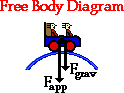Given Info:

 m = 621 kg v = 18.9 m/s R = 12.7 m

Find:

Fapp at top of hill

Step 3 of the suggested method would not apply to this problem since there are no forces directed "at angles" (that is, all the forces are either horizontally or vertically directed). Step 4 of the suggested method involves the determination of any known forces. In this case, the force of gravity can be determined from the equation Fgrav = m * g. So the force of gravity acting upon the 621-kg car is approximately 6086 N. Step 5 of the suggested method involves the calculation of the acceleration from the given values of the speed and the radius. Using the equation given in Lesson 1, the acceleration can be calculated as follows

a = v2 / R

a = (18.9 m/s)2 / (12.7 m)

a = 28.1 m/s2

Step 6 of the suggested method involves the determination of an individual force - the applied force. This will involve a two-step process: first the net force (magnitude and direction) must be determined; then the net force must be used with the free body diagram to determine the applied force. This two-step process is shown below.

 Fnet = m • a Fnet = (621 kg) • (28.1 m/s2, down) Fnet = 17467 N, down   As shown in FBD at right: Fapp = Fnet - Fgrav Fnorm = 11381 N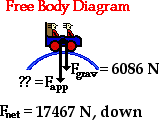Fapp and Fgrav must combine together (i.e., add up) to supply the required downwards net force of 17467 N.

This same method could be applied for any region of the track in which roller coaster riders momentarily experience circular motion.

### We Would Like to Suggest ...Sometimes it isn't enough to just read about it. You have to interact with it! And that's exactly what you do when you use one of The Physics Classroom's Interactives. We would like to suggest that you combine the reading of this page with the use of our Roller Coaster Model Interactive, our Roller Coaster Design Interactive, and/or our Barrel Ride Simulator. You can find these Interactives in the Physics Interactives section of our website. These Interactives allow a learner to interactively explore the physics principles that underly the safe design of a roller coaster.

.

### Check Your Understanding

1. Anna Litical is riding on The Shock Wave at Great America. Anna experiences a downward acceleration of 12.5 m/s2 at the top of the loop and an upward acceleration of 24.0 m/s2 at the bottom of the loop. Use Newton's second law to determine the normal force acting upon Anna's 50-kg body at the top and at the bottom of the loop.

2. Noah Formula is riding a roller coaster and encounters a loop. Noah is traveling 6 m/s at the top of the loop and 18.0 m/s at the bottom of the loop. The top of the loop has a radius of curvature of 3.2 m and the bottom of the loop has a radius of curvature of 16.0 m. Use Newton's second law to determine the normal force acting upon Noah's 80-kg body at the top and at the bottom of the loop.

3. Noah Formula is riding an old-fashioned roller coaster. Noah encounters a small hill having a radius of curvature of 12.0 m. At the crest of the hill, Noah is lifted off his seat and held in the car by the safety bar. If Noah is traveling with a speed of 14.0 m/s, then use Newton's second law to determine the force applied by the safety bar upon Noah's 80-kg body.

4. Anna Litical is riding a "woody" roller coaster. Anna encounters the bottom of a small dip having a radius of curvature of 15.0 m. At the bottom of this dip Anna is traveling with a speed of 16.0 m/s and experiencing a much larger than usual normal force. Use Newton's second law to determine the normal force acting upon Anna's 50-kg body.

Next Section: